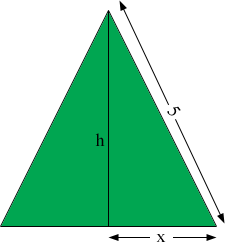SEARCH HOMEMath Central Quandaries & QueriesQuestion from valentin, a student: What is the maximum area of an isosceles triangle with two side lengths equal to 5 and one side length equal to 2x, where 0 ≤ x ≤ 5?Valentin,Use Pythagoras theorem to find the height h of the triangle. What is the area of the triangle? Use the calculus you know to maximize this expression.

If you have difficulty completing this write back and tell us where you are stuck and we will try to help.

HarleyMath Central is supported by the University of Regina and The Pacific Institute for the Mathematical Sciences.